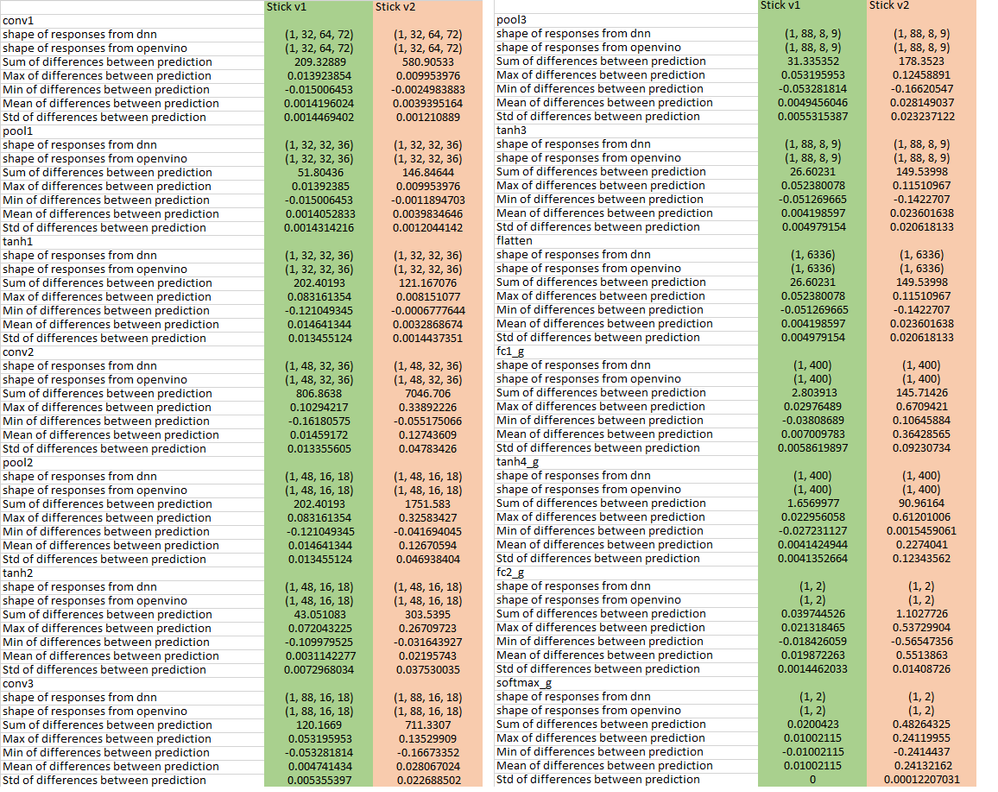Intel® Distribution of OpenVINO™ Toolkit
Community assistance about the Intel® Distribution of OpenVINO™ toolkit, OpenCV, and all aspects of computer vision-related on Intel® platforms.
6255 Discussions

## Incorrect network values when using movidius stick V2 and openvinoBeginner
198 Views

Hi, we have problems with the second stick model (the first version works fine). We run the same openvino-optimized model on two different versions of the device and get the following results (see attached image).
We compared dnn detector with openvino detector.
It seems that the error is growing fast enough, which gives unsatisfactory results of the network as a whole.

The keys for converting the model were used:
python mo.py --framework caffe --input_model <weights path> --input_proto <proto path> --output_dir <results folder>  --output layerName --disable_fusing --data_type FP16

Function for print table:

```def print_diff(layer, prediction_from_dnn, prediction_from_ov):
print(layer)
print('shape of responses from dnn             : ', prediction_from_dnn.shape)
print('shape of responses from openvino        : ', prediction_from_ov.shape)
print('Sum of differences between prediction   : ', np.sum(np.abs(prediction_from_dnn-prediction_from_ov)))
print('Max of differences between prediction   : ', np.max(prediction_from_dnn-prediction_from_ov))
print('Min of differences between prediction   : ', np.min(prediction_from_dnn-prediction_from_ov))
print('Mean of differences between prediction  : ', np.mean(np.abs(prediction_from_dnn-prediction_from_ov)))
print('Std of differences between prediction   : ', np.std(np.abs(prediction_from_dnn-prediction_from_ov)))
return 0```Can somebody comment this moment? Thanks!